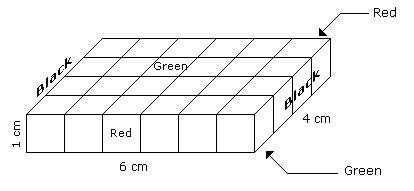# Verbal Reasoning - Cube and Cuboid

Exercise : Cube and Cuboid - Cube and Cuboid 1
Directions to Solve

The following questions are based on the information given below:

1. A cuboid shaped wooden block has 6 cm length, 4 cm breadth and 1 cm height.
2. Two faces measuring 4 cm x 1 cm are coloured in black.
3. Two faces measuring 6 cm x 1 cm are coloured in red.
4. Two faces measuring 6 cm x 4 cm are coloured in green.
5. The block is divided into 6 equal cubes of side 1 cm (from 6 cm side), 4 equal cubes of side 1 cm(from 4 cm side).

1.
How many cubes having red, green and black colours on at least one side of the cube will be formed ?
16
12
10
4
Explanation:Such cubes are related to the corners of the cuboid. Since the number of corners of the cuboid is 4.

Hence, the number of such small cubes is 4.

2.
How many small cubes will be formed ?
6
12
16
24
Explanation:Number of small cubes = l x b x h = 6 x 4 x 1 = 24

3.
How many cubes will have 4 coloured sides and two non-coloured sides ?
8
4
16
10
Explanation:Only 4 cubes situated at the corners of the cuboid will have 4 coloured and 2 non-coloured sides.

4.
How many cubes will have green colour on two sides and rest of the four sides having no colour ?
12
10
8
4
Explanation:There are 16 small cubes attached to the outer walls of the cuboid.

Therefore remaining inner small cubes will be the cubes having two sides green coloured.

So the required number = 24 - 16 = 8

5.
How many cubes will remain if the cubes having black and green coloured are removed ?
4
8
12
16